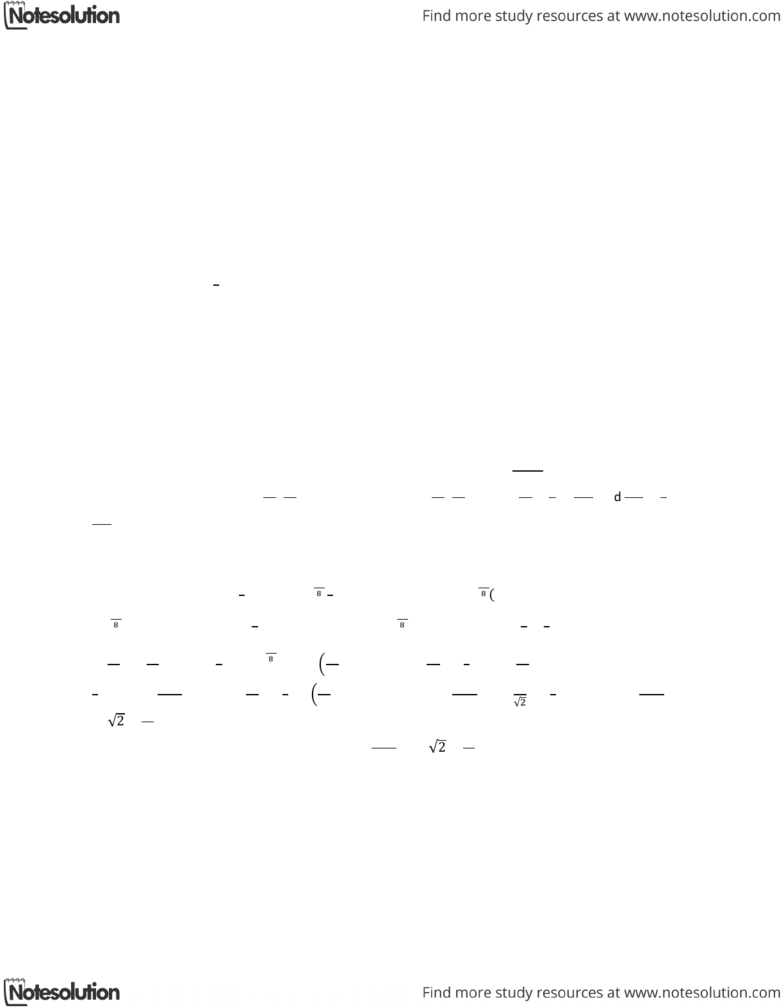Class Notes (1,100,000)
CA (630,000)
UTSG (50,000)
MAT (4,000)
MAT136H1 (900)
all (200)
Lecture

# MAT136H1 Lecture Notes - Polar Coordinate System, Strategy First

Department
Mathematics
Course Code
MAT136H1
Professor
all

Page:
of 110. Parametric Equations & Polar Coordinates
Area & Length in Polar Coordinates
Question #5 (Medium): Area Inside Two Polar Curves
Strategy
First the point of intersection need to be determined by setting the two polar equations equal to each
other. Then based on the symmetry the integral can be simplified. Based on the graph, detect which
polar equation sets the area that lies within both curves. Then use that polar equation to determine te
area base on  

Sample Question
Find the area of the region that lies inside both polar curves.
    ,
Solution
First the points of intersection need to be determined by setting the two polar equations equal to each
other:   . Then 4 can be dropped from both sides, then divided by 3 on both
sides. Then:   . Divide both sides by , then:   
  . Let  .
Then    means 

but since   , 

and also 

, and 

. But since the graph is symmetrical in both x-axis and y-axis direction, only a quarter can be set using
integral then multiplied by 4. Since it is the area that lies inside both curves, it is then  
upto the point of intersection.
Then the area is:  

 


   




 



 












 

Therefore the area that lies inside both curves is 
